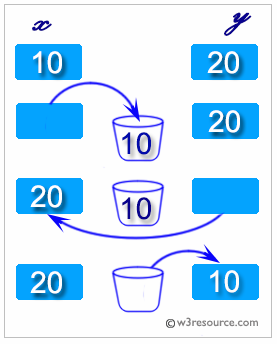﻿ Python: Swap two variables - w3resource# Python: Swap two variables

## Python Basic: Exercise-91 with Solution

Write a Python program to swap two variables.

Python: swapping two variables

Swapping two variables refers to mutually exchanging the values of the variables. Generally, this is done with the data in memory.

The simplest method to swap two variables is to use a third temporary variable :

```define swap(a, b)
temp := a
a := b
b := temp
```Sample Solution-1:

Python Code:

``````a = 30
b = 20
print("\nBefore swap a = %d and b = %d" %(a, b))
a, b = b, a
print("\nAfter swaping a = %d and b = %d" %(a, b))
print()
```
```

Sample Output:

```Before swap a = 30 and b = 20

After swaping a = 20 and b = 30
```

## Visualize Python code execution:

The following tool visualize what the computer is doing step-by-step as it executes the said program:

Sample Solution-2:

Python Code:

``````x = 34
y = 56
print("Initial Value of x =", x)
print("Initial Value of y =", y)
temp = x
x = y
y = temp
print("\nAfter swaping value of x =", x)
print("After swaping value of y =", y)
```
```

Sample Output:

```Initial Value of x = 34
Initial Value of y = 56

After swaping value of x = 56
After swaping value of y = 34
```

## Visualize Python code execution:

The following tool visualize what the computer is doing step-by-step as it executes the said program:

Python Code Editor:

Have another way to solve this solution? Contribute your code (and comments) through Disqus.

What is the difficulty level of this exercise?

Test your Python skills with w3resource's quiz

﻿

## Python: Tips of the Day

Try-catch-else construct:

```try:
foo()
except Exception:
print("Exception occured")
else:
print("Exception didnt occur")
finally:
print("Always gets here")
```